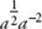﻿ ﻿THE NUMBER LINE - Basic Arithmetic - TOPICS IN ARITHMETIC - SAT SUBJECT TEST MATH LEVEL 1

## TOPICS IN ARITHMETIC## CHAPTER 2Basic Arithmetic• The Number Line

• Absolute Value

• Integers

• Exponents and Roots

• Squares and Square Roots

• Logarithms

• PEMDAS

• Exercises

To do well on the Math Level 1 test, you need a good working knowledge of arithmetic—not because there are several questions on arithmetic (in fact, there are very few) but because arithmetic is the basis for much of the math that is on the test. In theory, you do not have to know how to subtract negative numbers, how to add fractions, or what fractional and negative exponents mean because you can use your calculator to evaluate (–3) – (–5) andand. However, you must know the rules so that you can subtract (–3xy) – (–5xy) and addand simplify.

### THE NUMBER LINE

On the Math 1 test, one or two questions will involve i, the imaginary unit, which you will read about later in Chapter 17. Otherwise, the word number always means real number, a number that can be represented by a point on the number line.A positive number is a number that lies to the right of 0 on the number line. A negative number lies to the left of 0 on the number line.

Key Fact A1

TRICHOTOMY LAW

For any real number a, exactly one of the following statements is true:

a is negative

a = 0

a is positive

﻿